# A farmer's heritage

A farmer died leaving his 17 horses to his 3 sons.
When his sons opened up the Will it read:
My eldest son should get 1/2 (half) of total horses;
My middle son should be given 1/3rd (one-third) of the total horses;
My youngest son should be given 1/9th (one-ninth) of the total horses.

How many horses should each son-heir get?

a =  9
b =  6
c =  2

### Step-by-step explanation:

$b={n}_{2}\mathrm{/}3=18\mathrm{/}3=6$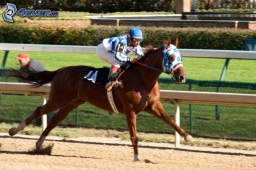Did you find an error or inaccuracy? Feel free to write us. Thank you!Tips to related online calculators
Need help to calculate sum, simplify or multiply fractions? Try our fraction calculator.

## Related math problems and questions:

• The king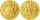The king divided ducats to his sons. He gave the eldest son a certain number of ducats, gave the younger one ducat less, gave the other one ducat less, and proceeded to the youngest. Then he returned to his eldest son, gave him one ducat less than a while
• Rajendra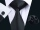Rajendra a farmer had two sons and two daughters. He decided to divide his property among his sons and daughters. So he wrote a "WILL" about distribution of his property. According to his "WILL", he desired to give 3/5 th of the property to his sons in eqOn the meadow grazing horses, cows, and sheep, together with less than 200. If cows were 45 times more, horses 60 times more, and sheep 35 times more than there are now, their numbers would equally. How many horses, cows, and sheep are on the meadow toget
• Sum of the seventeen numbersThe sum of the 17 different natural numbers is 154. Determine the sum of the two largest ones.
• NumbersDetermine the number of all positive integers less than 4183444 if each is divisible by 29, 7, 17. What is its sum?
• Meanif the mean of the set of data 5, 17, 19, 14, 15, 17, 7, 11, 16, 19, 5, 5, 10, 8, 13, 14, 4, 2, 17, 11, x is -91.74, what is the value of x?
• Salat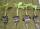Grandmother planted salad. In each row, he planted 13 seedlings. After a morning frost, many seedlings died. In the first row, five seedlings died. In the second row, two seedlings died more than 1st row. In the third row, three seedlings died less than 1
• Ravens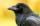The tale of the Seven Ravens were seven brothers, each of whom was born exactly 2.5 years after the previous one. When the eldest of the brothers was 2-times older than the youngest, mother all curse. How old was seven ravens brothers when their mother cu
• Three siblings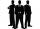Three siblings have birthday in one day-today. Together they have 35 years today. The youngest is three years younger than middle and the oldest is 5 years older than middle. How old is each?
• Gold inheritance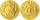The king divided the inheritance with his three sons in the ratio of 7: 6: 4. Two of them received 286,000 gold. How much each of the sons got.
• DiophantusWe know little about this Greek mathematician from Alexandria, except that he lived around 3rd century A. D. Thanks to an admirer of his, who described his life through an algebraic riddle, we know at least something about his life. Diophantus's youth las
• The inheritanceDad originally decided to bequeath the money to his two sons so that they would share it 8: 7 (older and younger). Then he changed his mind and divided the same amount in the ratio 11: 9 (older and younger). This increased the value of the inheritance for
• Ducats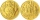The king divided the ducats to his three sons in a ratio of 2: 5: 4. How many ducats the king divided them if the youngest received 260 ducats, the least of all sons.
• Multiplicative inverse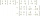Explain why the reciprocal of - 1 1/17 is the multiplicative inverse of - 17/18.
• Inheritance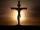After death father (♱ 62) remained mother (his wife) and its 3 children. Inheritance by law is that in the first mother will automatically get half of the property and other one-half inherited by heirs which are mother and her 3 children by the same share
• The agesThe ages of the four sons make an arithmetic sequence, the sum of which is the age of the father today. In three years, the father's age will be the sum of the ages of the three eldest sons, and in the next two years and three months, the father's age wil
• Farmer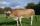The farmer farming 293 ha of arable land and the remaining 20% are meadows and pastures. How much land farmer owns?